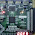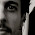Saturday, October 8, 2011

Circle Detection using OpenCV

Circle detection is an algorithm that detects any number of circles in a given image. OpenCV provides an efficient algorithm that makes use of Hough Transform. This article provides steps and information on how OpenCV detects circle and how to optimize Hough Transform parameters for better circle detection.

You Need
1. Plain, less textured (non-textured preferred) background.
2. Test object (Penny or Tennis Ball or simple bottle cap).
Code Snippet
Following is a code snippet that performs circle detection. Here frame is of IplImage that is captured from USB camera.

Here comes steps to be done for better circle detection.
• Input image should be of gray scale only. Use cvCvtColor to convert color scaled image to gray scale.
//Process Frame for circle detection
cvCvtColor(frame, gray, CV_BGR2GRAY);
• Perform Guassian smoothing using cvSmooth function with filter size as 9x9 to remove noises or any sharp edges. Filter size should be of odd number.
//Perform Gaussian smoothing to reduce sharp edges and noise.
cvSmooth(gray, gray, CV_GAUSSIAN, 9, 9);
• Detect circle using cvHoughCircles function by passing gray scale input image. Syntax of cvHoughCircles is given as:
CvSeq* cvHoughCircles(CvArr* image, CvMemStorage* circleStorage, int method, double dp, double minDist, double param1=100,  double param2=100, int minRadius=0, int maxRadius=0 )
For parameters explanation, check out here.

//Detect circles in left frame.
circles = cvHoughCircles(gray, storage, CV_HOUGH_GRADIENT, 2, 20, 100, 155, 20, 300);
• cvHoughCircles returns number of detected circles.
• Next step is to draw detected circle using cvCircle function. In above code snippet, l,l defines x,y coordinate of detected circle centre. l defines radius of detected circle.
for(i = 0; (circles-> total)  >= 2 ? i  < 1 : i < circles->total; i++)
{
l = (float*)cvGetSeqElem(circles, i);
//Draw detected Circle border.
cvCircle(frame, cvPoint(cvRound(l),cvRound(l)), cvRound(l), CV_RGB(255,0,0), 3, 8, 0 );
}

Tuning cvHoughCircles
With improper tuning of Hough Transform parameter, either more number of circles are drawn or none of circles are detected. So you have to wisely tune parameters for better result.

- minDist parameter defines minimum distance between circles that has to be detected. If this is too large, circles are '''missed'''. If small, more number of unwanted circles are drawn.
- param1 should be 3/4th lesser than param2. Else no circles will be detected.
- minRadius defines minimum radius of circles to be detected. This can be zero for non-textured background.
- maxRadius defines maximum radius of circle to detect.

By proper tuning of these parameters, objects like coins, ball can be detected well. The main drawback and time consuming part is trial and error of tuning Hough Transform parameters and if improper values are choosen, this transform detects irrelevant non circled area. But once properly tuned, you can play with it to do any operation like depth calculation of object from camera.

Above image shows coins being detected using Hough Transform. Your comments are welcomed:-)  Have Fun!!!

1.Hi,
I tried this hough transform for detecting iris but it doesn help. It detects circle only wen d transition is high. In my image , i have eye on black background. so it detects only the outer circle. can u plz let me know if thr s any other way to detect circle.

2.is there any way to find the area or diameter of detected circles?????

3.Hi Syed,

Yes, you will receive the radius of the detected circle from cvHoughCircles function. From this you can calculate diameter and area of detected Circle.

SathyaKumar P

4.Hello, thanks for your post....
Tuning cvHoughCircles you said "...aram1 should be 3/4th lesser than param2..."

Why?